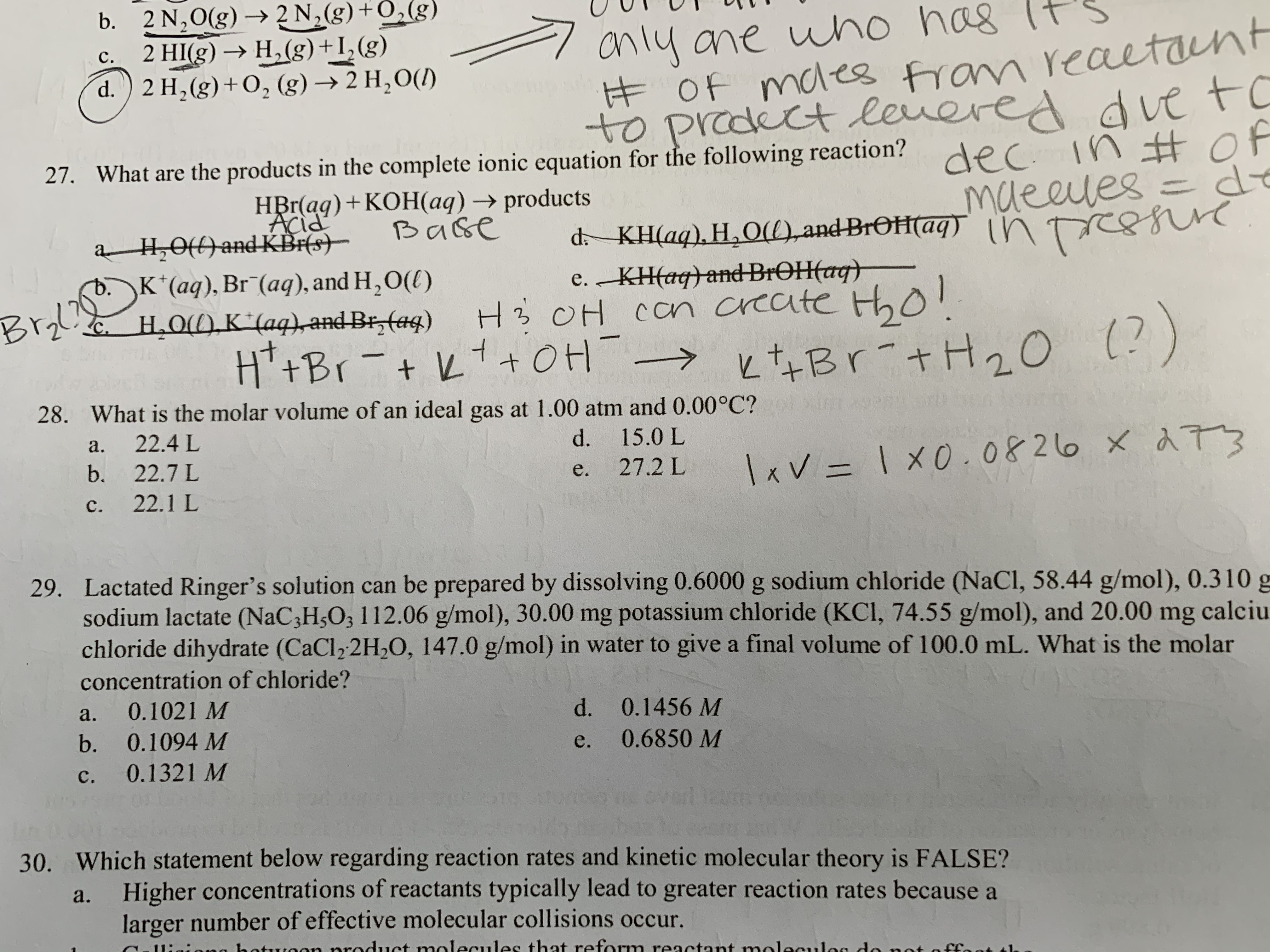# b. 2 N,0(g) → 2 N,(g)+0,(g)2 HI(g) → H,(g)+I,(g)anly ane who has It'sĦOF mdes fran reaetanted dve toc.d.) 2 H, (g)+O, (g) → 2 H,O(1)to prodect leveredec.27. What are the products in the complete ionic equation for the following reaction? in Hofdemaeeles=ressiuc.HBr(ag)+KOH(aq) → productsBaseAcida H,0() and-KBr(s)–b.K*(aq), Br (aq), and H,O(()Brol H.O1), K*(aq),andBr, (aq)d- KH(aq), H,O(),and BrOH(aq) intoe. KH(aq}and-BROH(ag)H3 OH con create Hho!H*+Br-+ L+OH → とtなBr +H20 )Bryl?(?)28. What is the molar volume of an ideal gas at 1.00 atm and 0.00°C?a.22.4 Ld. 15.0 Lb.Ixv = 1 x0.0826 x 2 7322.7 Le.27.2 LC.22.1 L29. Lactated Ringer's solution can be prepared by dissolving 0.6000 g sodium chloride (NaCl, 58.44 g/mol), 0.310 gsodium lactate (NaC3H5O; 112.06 g/mol), 30.00 mg potassium chloride (KCI, 74.55 g/mol), and 20.00 mg calciuchloride dihydrate (CaCl2 2H2O, 147.0 g/mol) in water to give a final volume of 100.0 mL. What is the molarconcentration of chloride?0.1021 Md. 0.1456 Ma.b.0.1094 M0.6850 Me.C.0.1321 Movarl zum30. Which statement below regarding reaction rates and kinetic molecular theory is FALSE?Higher concentrations of reactants typically lead to greater reaction rates because alarger number of effective molecular collisions occur.a.

Question
1 viewshelp_outlineImage Transcriptioncloseb. 2 N,0(g) → 2 N,(g)+0,(g) 2 HI(g) → H,(g)+I,(g) anly ane who has It's ĦOF mdes fran reaetant ed dve to c. d.) 2 H, (g)+O, (g) → 2 H,O(1) to prodect levere dec. 27. What are the products in the complete ionic equation for the following reaction? in Hof de maeeles= ressiuc. HBr(ag)+KOH(aq) → products Base Acid a H,0() and-KBr(s)– b.K*(aq), Br (aq), and H,O(() Brol H.O1), K*(aq),andBr, (aq) d- KH(aq), H,O(),and BrOH(aq) into e. KH(aq}and-BROH(ag) H3 OH con create Hho! H*+Br-+ L+OH → とtなBr +H20 ) Bryl? (?) 28. What is the molar volume of an ideal gas at 1.00 atm and 0.00°C? a. 22.4 L d. 15.0 L b. Ixv = 1 x0.0826 x 2 73 22.7 L e. 27.2 L C. 22.1 L 29. Lactated Ringer's solution can be prepared by dissolving 0.6000 g sodium chloride (NaCl, 58.44 g/mol), 0.310 g sodium lactate (NaC3H5O; 112.06 g/mol), 30.00 mg potassium chloride (KCI, 74.55 g/mol), and 20.00 mg calciu chloride dihydrate (CaCl2 2H2O, 147.0 g/mol) in water to give a final volume of 100.0 mL. What is the molar concentration of chloride? 0.1021 M d. 0.1456 M a. b. 0.1094 M 0.6850 M e. C. 0.1321 M ovarl zum 30. Which statement below regarding reaction rates and kinetic molecular theory is FALSE? Higher concentrations of reactants typically lead to greater reaction rates because a larger number of effective molecular collisions occur. a. fullscreen
check_circle

Step 1

Since molar volume means volume of 1 mole of gas.

hence n = 1  = moles

And P = 1 atm

And T = ...

### Want to see the full answer?

See Solution

#### Want to see this answer and more?

Solutions are written by subject experts who are available 24/7. Questions are typically answered within 1 hour.*

See Solution
*Response times may vary by subject and question.
Tagged in